﻿

### Lifting quasifribations

#### Abstract

The construction of "lifting" spreads in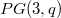$PG(3, q)$ to spreads in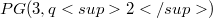$PG(3, q2)$ is generalized to lifting quasifibrations in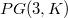$PG(3,K)$ to quasifibrations in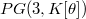$PG(3, K[\theta])$ for a quadratic extension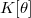$K[\theta]$ of a field K.

DOI Code: 10.1285/i15900932v16n1p25

Full Text: PDF